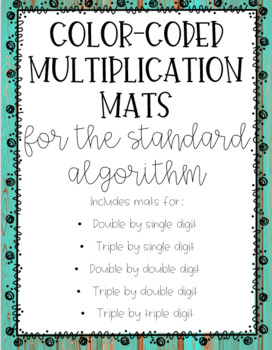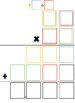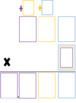# Color Coded Multiplication MatsSubject
Resource Type
File Type

Zip

(1 MB|22 pages)
Product Rating
4.0
(1 Rating)
Standards
• Product Description
• StandardsNEW

These multiplication mats are great for small group or intervention work!

It is best used with students who know the order to multiply with the standard algorithm, but are having difficulty knowing when to exchange ones for tens and where to place the products. The color coded system allows students to practice the proper placement so that the algorithm becomes natural.

It can also be used with students who already know how to multiply with the standard algorithm for centers using dice to create the numbers.

The product comes with ready to print mats and PNG files that can be inserted into Paint, PowerPoint, Smart Notebook, or OneNote so students can use the draw feature to solve the problem using the mat. The second option does not require printing in color.

To use, students will multiply the numbers in the order of place value following the standard algorithm. Then, they will use the colors to decide where each digit in the partial product will go.

For instance, the ones digit of the blue product will go in the ones blue box while the tens digit will go in the second blue box over the tens place.

The gold plus sign is next to this blue box to remind the student to add that number after the gold numbers are multiplied.

There are a set of completed multiplication problems using each mat included in the product. Each set includes a problem that uses only some of the available boxes while the other uses all the boxes to solve.

Includes mats for:

•Double by single digit

•Triple by single digit

•Double by double digit

•Triple by double digit

•Triple by triple digit

Please review! I would love to hear your thoughts, opinions, or how you use it in your classroom!

Fluently multiply multi-digit whole numbers using the standard algorithm.
Multiply a whole number of up to four digits by a one-digit whole number, and multiply two two-digit numbers, using strategies based on place value and the properties of operations. Illustrate and explain the calculation by using equations, rectangular arrays, and/or area models.
Multiply one-digit whole numbers by multiples of 10 in the range 10–90 (e.g., 9 × 80, 5 × 60) using strategies based on place value and properties of operations.
Total Pages
22 pages
N/A
Teaching Duration
N/A
Report this Resource to TpT
Reported resources will be reviewed by our team. Report this resource to let us know if this resource violates TpT’s content guidelines.